Thursday, May 31, 2018

(x, why?) Mini: Making Toast

(Click on the comic if you can't see the full image.)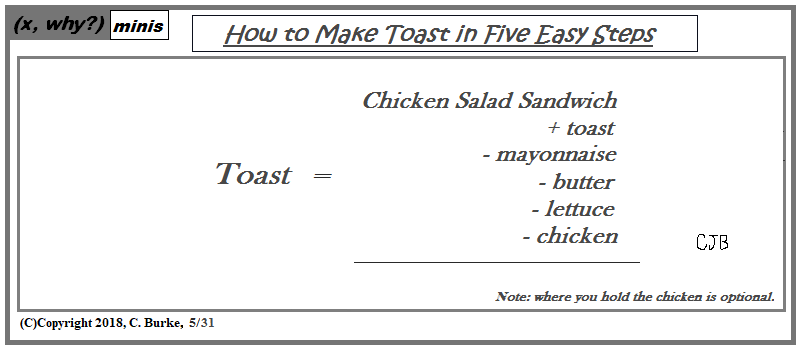The equation doesn't break any rules.
Suggested from the Cheese Stands Alone strip, except this comic doesn't really solve for toast, as toast occurs on both sides of the equation.

Come back often for more funny math and geeky comics.Wednesday, May 30, 2018

Algebra 2 Problems of the Day

Daily Algebra 2 questions and answers.

More Algebra 2 problems.

June 2017, Part I

All Questions in Part I are worth 2 credits. No work need be shown. No partial credit.

1. The graph of the function p(x) is sketched below.

Which equation could represent p(x)?
1) p(x) = (x2 − 9)(x − 2)
2) p(x) = x3 − 2x2 + 9x + 18
3) p(x) = (x22 + 9)(x − 2)
4) p(x) = x3 + 2x2 − 9x − 18

Answer: 1) p(x) = (x2 − 9)(x − 2)
The zeroes are -3, 2, and 3, so the factors are (x + 3)(x - 2)(x - 3).
(x + 3)(x - 3) = x2 - 9.
Choice 3 is bad because it has x2 + 9, which doesn't have zeroes.
Choice 4 is bad because (3)(-2)(-3) = 18, not -18.

2. What is the solution to 8(2x + 3) = 48?
1) x = ln6/ln2 - 3
2) x = 0
3) x = ln48/ln16 - 3
4) x = ln4 - 3

Answer: 1) x = ln6/ln2 - 3

8(2x + 3) = 48
2x + 3 = 6
(x + 3)ln 2 = ln 6
x + 3 = ln6/ln2
x = ln6/ln2 - 3

3. Cheap and Fast gas station is conducting a consumer satisfaction survey. Which method of collecting data would most likely lead to a biased sample?
1) interviewing every 5th customer to come into the station
2) interviewing customers chosen at random by a computer at the checkout
3) interviewing customers who call an 800 number posted on the customers' receipts
4) interviewing every customer who comes into the station on a day of the week chosen at random out of a hat

Answer: 3) interviewing customers who call an 800 number posted on the customers' receipts
Self-selection introduces bias because those with stronger opinions are more likely to call the number. You would not get a random sample.

More Algebra 2 problems.

Tuesday, May 29, 2018

(x, why?) Mini: Length & Width

(Click on the comic if you can't see the full image.)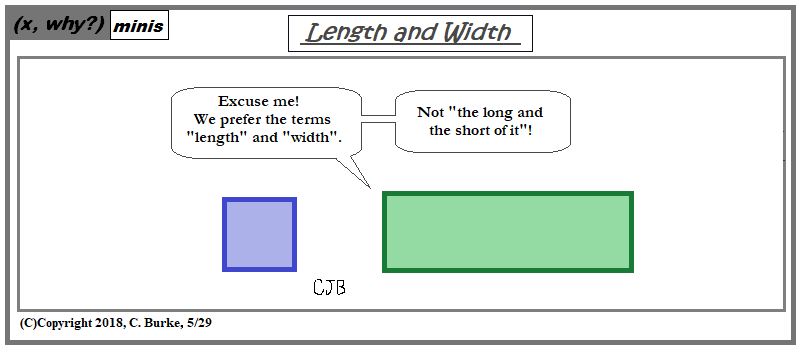Don't get them started on the whole base and height thing.

Come back often for more funny math and geeky comics.Monday, May 28, 2018

Memorial Day 2018

(Click on the comic if you can't see the full image.)To walk among the heroes is a humbling experience. And, yes, I can't help notice the lines stretching out in each direction, even as I move.

Come back often for more funny math and geeky comics.Sunday, May 27, 2018

Comic #1313

(Click on the comic if you can't see the full image.)I wanted to work in a Mockingbird Lane reference, or at least Nevermore, but I couldn't construct it properly and give the pun life.

Note to self: next time I take a photo for a comic, don't zoom in so much that I have little room for word balloons.

Come back often for more funny math and geeky comics.Friday, May 25, 2018

Peace-wise

(Click on the comic if you can't see the full image.)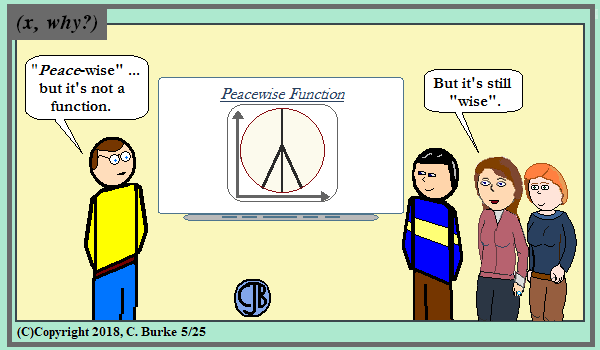Before you ask, it also can't be represented as piecewise. It's a system of equations: an absolute value and a vertical line. It's less clear if the circle is graphed or added for emphasis. Who can figure out what Ken is thinking?

Then again, who can figure out what Michele is thinking? She's the one marrying him!

Come back often for more funny math and geeky comics.Thursday, May 24, 2018

(x, why?) Mini: The Cheese Stands Alone

(Click on the comic if you can't see the full image.)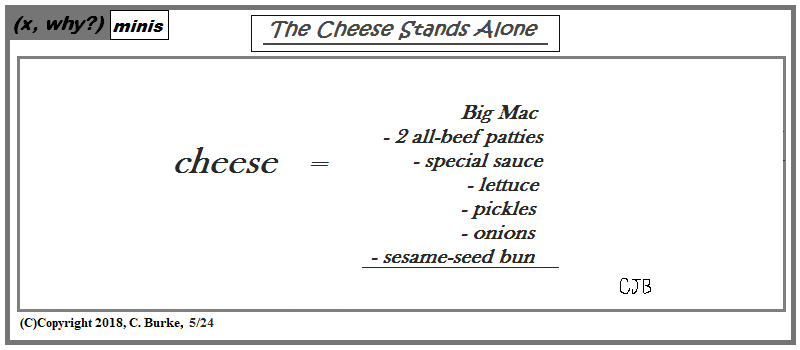To isolate the cheese, you need to hold the pickles, hold the lettuce, hold everything else and maybe switch franchises.
At least you don't have to hold the chicken.

I debated whether or not to use an actual trademarked property, but I didn't want to use the word cheese on the right side of the equation.

Come back often for more funny math and geeky comics.Algebra 2 Problems of the Day (open ended)

Daily Algebra 2 questions and answers.

More Algebra 2 problems.

August 2017, Part IV

The Question in Part IV is worth 6 credits. Work need be shown (or explained or justified) for full credit. Correct numerical answers with no work receive one credit.

37. The value of a certain small passenger car based on its use in years is modeled by V(t) = 28482.698(0.684)t, where V(t) is the value in dollars and t is the time in years. Zach had to take out a loan to purchase the small passenger car. The function Z(t) = 22151.327(0.778)t, where Z(t) is measured in dollars, and t is the time in years, models the unpaid amount of Zach’s loan over time.

Graph V(t) and Z(t) over the interval 0 < t < 5, on the set of axes below.

State when V(t) = Z(t), to the nearest hundredth, and interpret its meaning in the context of the problem.

Zach takes out an insurance policy that requires him to pay a \$3000 deductible in case of a collision. Zach will cancel the collision policy when the value of his car equals his deductible. To the nearest year, how long will it take Zach to cancel this policy? Justify your answer.

Create Tables of Values for both V(t) and Z(t). You will see that an interval of 0 to 5 will be sufficient. Next, determine the scale. There is enough room on the y-axis for the scale to be 1 box to equal \$2,000. The x-axis will measure the time, and you can use every 2, 3 or 4 boxes for each year (be consistent!) to spread the graph out, for readability.
The plot the points and label the graphs and the axes.
See the images below.

The point where V(t) = Z(t) can be found be finding the point of intersection in your calculator. This happens at about 1.95233, or 1.95, to the nearest hundredth. In the context of the problem, this is the point where the value of the car is equal to the remaining balance of the loan.

For the final portion of the question, since they want the answer rounded the nearest year, you don't need to solve algebraically. You only need to continue the table and find when the car will be worth less than \$3000. At year 6, the car's value is V(6) = 28482.698(0.684)6 = 2916.87.
It is reasonable after six years, because that is the first time that the car will be worth less than \$3000.

More Algebra 2 problems.

Monday, May 21, 2018

Unit Analysis

(Click on the comic if you can't see the full image.)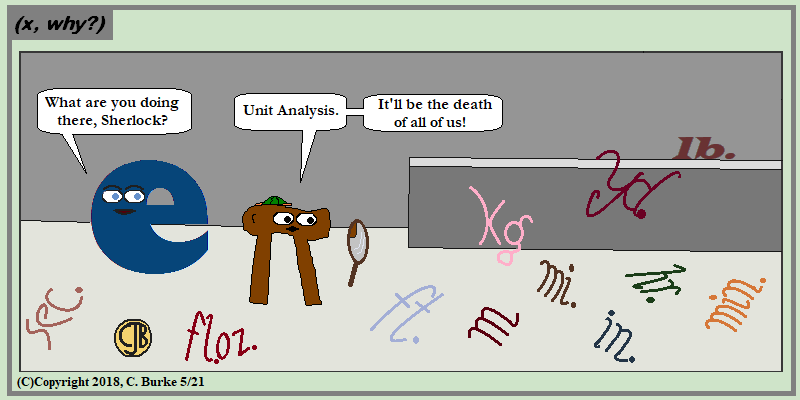'Wizard of floz' or 'floz after every meal'? Which should I use?

Come back often for more funny math and geeky comics.Algebra 2 Problems of the Day (open ended)

Daily Algebra 2 questions and answers.

More Algebra 2 problems.

August 2017, Part III

All Questions in Part III are worth 4 credits. Work need be shown (or explained or justified) for full credit. Correct numerical answers with no work receive one credit.

35.a) On the axes below, sketch at least one cycle of a sine curve with an amplitude of 2, a midline at y = -3/2 and a period of 2π.

b) Explain any differences between a sketch of y = 2 sin (x - π/3) - 3/2 and the sketch from part a.

The sine curve starts at the midline value. In y = sin x, the midline would be zero, and it would start at the origin. This curve will start at (0, -3/2). The amplitude is 2, so it has a maximum at -3/2 + 2 = 0.5 (or 1/2), and a minimum at -3/2 - 2 = -3.5 (or -7/2). Label the graph!

They didn't ask for it, but you can create the equation from the description above. (This may also be useful if you wish to use your calculator.)
With an amplitude of 2, period of 2π, and midline of y = -3/2, the sine curve would be

y = 2 sin x - 3/2

See the image below.

In part b, the only difference between the original curve and the new curve is the "- π/3" in the parentheses. This represents a translation of the original curve.
The original sketch will shift π/3 units to the right.

36. Using a microscope, a researcher observed and recorded the number of bacteria spores on a large sample of uniformly sized pieces of meat kept at room temperature. A summary of the data she recorded is shown in the table below.

Using these data, write an exponential regression equation, rounding all values to the nearest thousandth.

The researcher knows that people are likely to suffer from food-borne illness if the number of spores exceeds 100. Using the exponential regression equation, determine the maximum amount of time, to the nearest quarter hour, that the meat can be kept at room temperature safely

Put the data into lists L1 and L2 in your calculator and do an exponential regression. You will get, to the nearest thousandth:
y = 4.168(3.981)x

In part b, substitute 100 for y and solve for x.

100 = 4.168(3.981)x
100/4.168 = (3.981)x
23.99232 = (3.981)x
log (23.99232) = log (3.981)x
log (23.99232) = x log (3.981)
log (23.99232) / log (3.981) = x
x = 2.300...

The nearest quarter hour is 2 1/4 hours or 2 hours and 15 minutes.

More Algebra 2 problems.

Sunday, May 20, 2018

Algebra 2 Problems of the Day (open ended)

Daily Algebra 2 questions and answers.

More Algebra 2 problems.

August 2017, Part III

All Questions in Part III are worth 4 credits. Work need be shown (or explained or justified) for full credit. Correct numerical answers with no work receive one credit.

33. Solve for all values of p:

3p / (p - 5) - 2 / (p + 3) = p / (p + 3)

Move the fraction with the (p + 3) denominator to the right side and combine the fractions. Next cross-multiply to get a quadratic equation. Set the equation equal to 0 by moving everything to the left side. Divide the equation by 2 to get rid of the leading coefficient. The remaining trinomial is ridiculously easy to factor into (p + 5) and (p - 1). That means that the solutions are p = -5 and p = -1.
According to original equation, +5 and -3 would have to be rejected as solutions. However, -5 is fine, so do not reject it (or -1, for that matter).

Refer to the image below:

34. Simon lost his library card and has an overdue library book. When the book was 5 days late, he owed \$2.25 to replace his library card and pay the fine for the overdue book. When the book was 21 days late, he owed \$6.25 to replace his library card and pay the fine for the overdue book.
Suppose the total amount Simon owes when the book is n days late can be determined by an arithmetic sequence. Determine a formula for an, the nth term of this sequence.

The difference between the 5th term and the 21st term is \$4.00 over 21-5 = 16 days. That's a constant different of \$.25 per day.
Using the formula an = a1 + (n-1)d, we can find the fine for the first day
2.25 = a1 + 4(.25)
2.25 = a1 + 1.00
1.25 = a1 is the first day.
an = 1.25 + (n - 1)(.25)

To find the cost on the 60th day, substitute 60 for n:
an = 1.25 + (60 - 1)(.25) = 16.00
The fine will be \$16.00

More Algebra 2 problems.

Using Sine!

(Click on the comic if you can't see the full image.)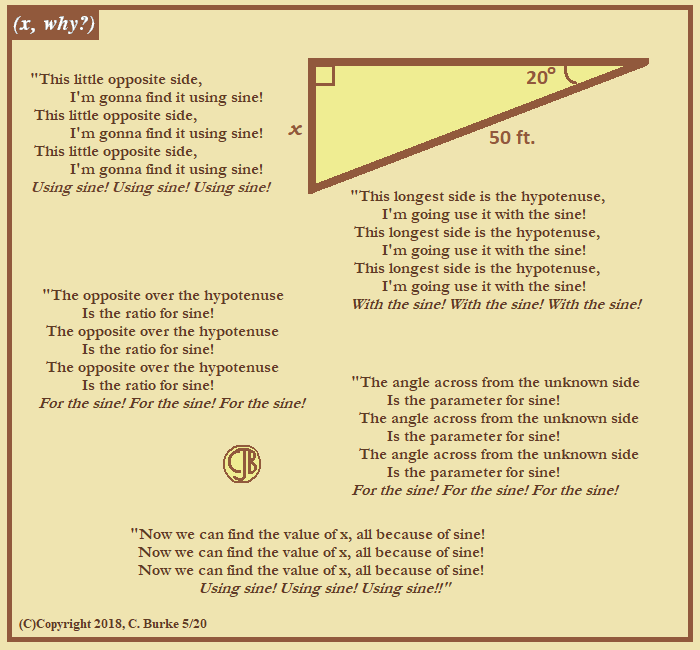Come back often for more funny math and geeky comics.Friday, May 18, 2018

Algebra 2 Problems of the Day (open ended)

Daily Algebra 2 questions and answers.

More Algebra 2 problems.

August 2017, Part II

All Questions in Part II are worth 2 credits. Work need be shown (or explained or justified) for full credit. Correct numerical answers with no work receive one credit.

31. Algebraically determine whether the function j(x)= x4 - 3x2 - 4 is odd, even, or neither.

A function is even if it is symmetrical about the y-axis. If reflected across the y-axis, it will be mapped onto itself. Because of this symmetry, for any value of x, f(x) = f(-x).
A function is odd if it is rotational symmetrical about the origin. If rotated 180 degrees about the origin, it will be mapped onto itself. Because of this symmetry, for any value of x, f(-x) = -f(x).

All the exponents (including the constant) have even exponents, so we know that the function is even. We need to show it algebraically, but knowing this tells you which situation you should start with.

f(-x) = (-x)4 - 3(-x)2 - 4
f(-x) = x4 - 3x2 - 4
f(-x) = f(x), therefore f(x) is even.

32. On the axes below, sketch a possible function p(x) = (x - a)(x - b)(x + c), where a, b, and c are positive, a > b, and p(x) has a positive y-intercept of d. Label all intercepts.

In the given function, a and b are roots on the right side of the y-axis (positive), and -c will be on left side (negative). Also, b comes before a because a > b. Finally, d is on the y-axis somewhere above the x-axis. This is just a sketch, it doesn't have to be perfect. At a minimum, please make sure your sketch passes the vertical line test -- don't be sloppy.

The line has to go from -c to d, so it starts in Quadrant III, through -c to d, then down to b and back up through a and beyond.
See the image below.
Note: d does not have to be a local maximum, but it could be. And c is a positive number, so the axis must be labeled -c.

More Algebra 2 problems.

Thursday, May 17, 2018

Algebra 2 Problems of the Day (open ended)

Daily Algebra 2 questions and answers.

More Algebra 2 problems.

August 2017, Part II

All Questions in Part II are worth 2 credits. Work need be shown (or explained or justified) for full credit. Correct numerical answers with no work receive one credit.

29. While experimenting with her calculator, Candy creates the sequence 4, 9, 19, 39, 79, … .
Write a recursive formula for Candy’s sequence.
Determine the eighth term in Candy’s sequence.

If you look at the differences, you see that the sequence increases by 5, then 10, then 20, then 40, etc. It does not have a common difference, but it does have a common ratio of 2, so it's a geometric sequence.
The initial value is 4, and 4 times 2 is 8, so you have to add another 1 to get 9, the second number in the sequence.

The recursive formula for this sequence will be:

a1 = 4
an = an-1 + 1

To get the 8th term in the sequence, just continue where the question left off. You were given the first 5 terms.
a6 = a5 + 1 = 2(79) + 1 = 159
a7 = a6 + 1 = 2(159) + 1 = 319
a8 = a7 + 1 = 2(319) + 1 = 639

30. In New York State, the minimum wage has grown exponentially. In 1966, the minimum wage was \$1.25 an hour and in 2015, it was \$8.75. Algebraically determine the rate of growth to the nearest percent.

2015 - 1966 = 49 years, which will be the exponent.
8.75 = 1.25(x)49
7 = x49
(7)(1/49) = x --> Yes, you are going to take the 49th root of 7.
x = 1.04051.., which is approximately 104%
This is about 4% growth to the nearest percent.

(Don't stop at 1.04, or round that to 1)

More Algebra 2 problems.

Octillion

(Click on the comic if you can't see the full image.)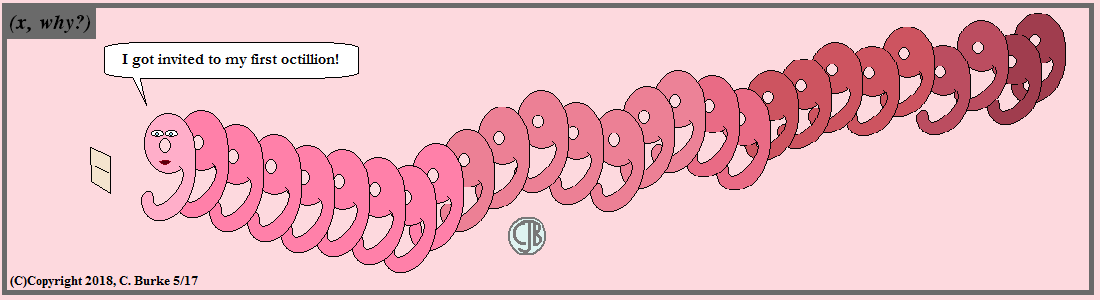I skipped the 'what to wear' joke -- you figure she'll round up something.

Come back often for more funny math and geeky comics.Tuesday, May 15, 2018

Algebra 2 Problems of the Day (open ended)

Daily Algebra 2 questions and answers.

More Algebra 2 problems.

August 2017, Part II

All Questions in Part II are worth 2 credits. Work need be shown (or explained or justified) for full credit. Correct numerical answers with no work receive one credit.

27. Verify the following Pythagorean identity for all values of x and y: (x2 + y2)2 = (x2 − y2)22 + (2xy)2

(x2 + y2)2 = (x2 − y2)22 + (2xy)2
x4 + 2x2y2 + y4 = x4 - 2x2y2 + y4 + 4x2y2
x4 + 2x2y2 + y4 = x4 + 2x2y2 + y4
(check mark!)

As long as you showed the left side and right side to be equal, you should be fine.
You could have only done the right side, leaving the left side as (x2 + y2)2, and as a final step, reduce the right side to (x2 + y2)2 as well.

28. Mrs. Jones had hundreds of jelly beans in a bag that contained equal numbers of six different flavors. Her student randomly selected four jelly beans and they were all black licorice. Her student complained and said "What are the odds I got all of that kind?" Mrs. Jones replied, "simulate rolling a die 250 times and tell me if four black licorice jelly beans is unusual." Explain how this simulation could be used to solve the problem.

A die has six sides, each of which is just as likely to occur. Each of the six sides could represent a color of a jelly bean in the bag. If, for example, 1, represents "black licorice", you could use the simulation to see how many times that this number would be rolled 4 times in a row after 250 simulations.

Make sure that you explain the simulation for full credit.

More Algebra 2 problems.

The Refrigerator

(Click on the comic if you can't see the full image.)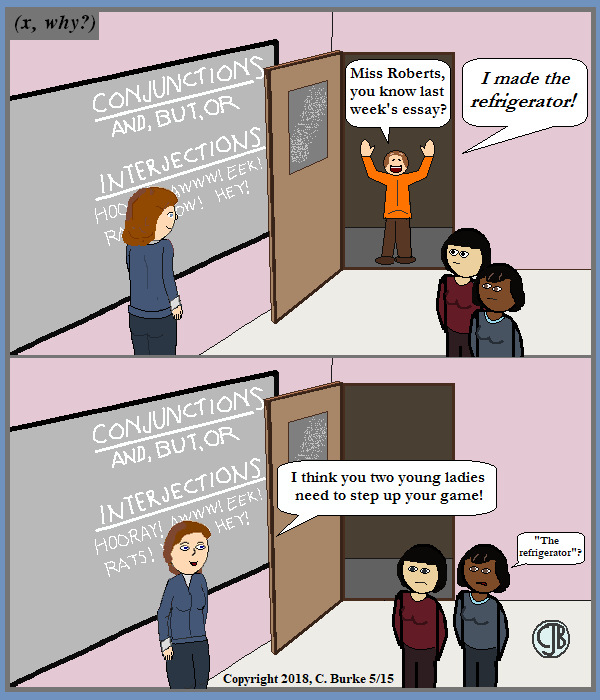Those fridge magnets have to hold up something. Just wait until you learn about magnets in Science!

That's Hank in the doorway. The two young ladies are new. Names pending. (Suggestions?)

Come back often for more funny math and geeky comics.Monday, May 14, 2018

Algebra 2 Problems of the Day (open-ended)

Daily Algebra 2 questions and answers.

More Algebra 2 problems.

August 2017, Part II

All Questions in Part II are worth 2 credits. Work need be shown (or explained or justified) for full credit. Correct numerical answers with no work receive one credit.

25.Explain how (−8)(4/3) can be evaluated using properties of rational exponents to result in an integer answer

A rational exponent, like (4/3), can be re-written as (4) * (1/3), which means to take the fourth power of the cube root of the expression (-8). (The power of a power rule)
The cube root of -8 is -2, which when raised to the 4th power is (-2)4 = 16, which is an integer.

26. A study was designed to test the effectiveness of a new drug. Half of the volunteers received the drug. The other half received a sugar pill. The probability of a volunteer receiving the drug and getting well was 40%. What is the probability of a volunteer getting well, given that the volunteer received the drug?

The probability of two things occurring is 40%. The combined probability can be found by multiplying the two independent probabilities.
Since we know one of those probabilities, we can solve for the other.
P(A) * P(B) = .40
1/2 * P(B) = .40
P(B) = .80
There was an 80% chance that if the volunteer received the drug that he (or she) would get better.

More Algebra 2 problems.

Sunday, May 13, 2018

Happy Mothers Day 2018

(Click on the comic if you can't see the full image.)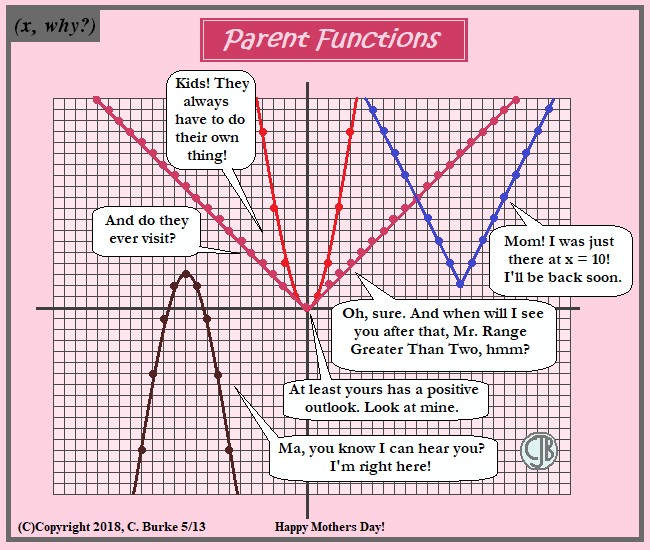Worry is a function of motherhood.

As for those transformations, if the parent function f(x) = x2 is transformed into g(x), then g(x) = -(x + 11)2 + 3

If the parent function h(x) = |x| is transformed into j(x), then j(x) = 2|x - 14)2 + 2

(I realize that I haven't been doing Mothers Day for a while. I hope you can understand.)

Come back often for more funny math and geeky comics.Thursday, May 10, 2018

Algebra 2 Problems of the Day

Daily Algebra 2 questions and answers.

More Algebra 2 problems.

August 2017, Part I

All Questions in Part I are worth 2 credits. No work need be shown. No partial credit.

22. A public opinion poll was conducted on behalf of Mayor Ortega's reelection campaign shortly before the election. 264 out of 550 likely voters said they would vote for Mayor Ortega; the rest said they would vote for his opponent.
Which statement is least appropriate to make, according to the results of the poll?

1) There is a 48% chance that Mayor Ortega will win the election.
2) The point estimate (ˆp) of voters who will vote for Mayor Ortega is 48%.
3) It is most likely that between 44% and 52% of voters will vote for Mayor Ortega.
4) Due to the margin of error, an inference cannot be made regarding whether Mayor Ortega or his opponent is most likely to win the election.

Answer: There is a 48% chance that Mayor Ortega will win the election.
Analysis of data. While 48% of the likely voters in this poll said that they would vote for Mayor Ortega, this is only a sample of all the voters and there is a margin of error. It is more appropriate to say that the point estimate is 48% than to say that this is his chance of winning.

23. What does ( (-54x9) / y4 )2/3 equal?

Check your work against the image below.
We need to take the cube root of the expression. We can factor -54 into (-27)(2) because -27 is a perfect cube. The cube root of x9 is x3. The y4 term can be broken into y3 times y, and the cube root of y3 is y.
After that we can square all the terms. (You could have done that sooner, if you don't mind squaring -54 and then finding the largest perfect cube that's a factor.)
(-3x3) squared is 9x6, over y2, times the cube root of 4 over y2. Split up the radical into a fraction of two radicals and you have choice 4.

The Rickerts decided to set up an account for their daughter to pay for her college education. The day their daughter was born, they deposited \$1000 in an account that pays 1.8% compounded annually. Beginning with her first birthday, they deposit an additional \$750 into the account on each of her birthdays. Which expression correctly represents the amount of money in the account n years after their daughter was born?

1) an = 1000(1.018)n + 750
2) an = 1000(1.018)n + 750n
3) a0 = 1000; an = an - 1 (1.018) + 750
4) a0 = 1000; an = an - 1 (1.018) + 750n

Answer:3) a0 = 1000; an = an - 1 (1.018) + 750
The initial value was \$1000. Each year the value of the account will by 1.8% greater plus another \$750 will be added to the balance. So the previous value, an - 1, times (1.018) then add 750.

Choice 1 doesn't work because the \$750 is only added once, instead of every year, and it isn't earning any interest.
Choice 2 doesn't work because the \$750, while being added each year, isn't earning the 1.8% interest.
Choice 4 shows a recursive formula, so no variable is needed at \$750. The parents aren't adding, for example, \$1500 on her second birthday or \$3000 on her fourth.

More Algebra 2 problems.

Wednesday, May 09, 2018

Sinusoidal

(Click on the comic if you can't see the full image.)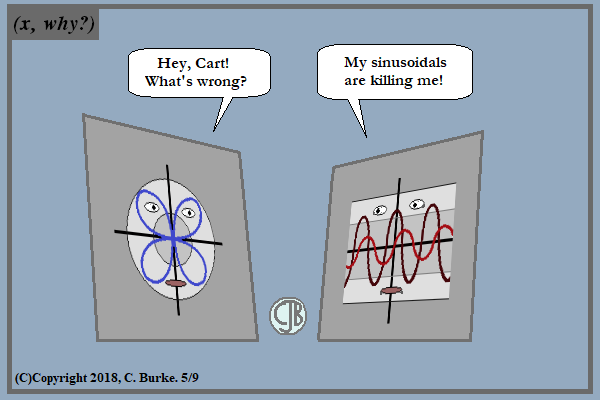Well, sure! We went straight from cold season to allergy season this year!

Come back often for more funny math and geeky comics.Tuesday, May 08, 2018

Algebra 2 Problems of the Day

Daily Algebra 2 questions and answers.

More Algebra 2 problems.

August 2017, Part I

All Questions in Part I are worth 2 credits. No work need be shown. No partial credit.

19. Consider the system shown below.

2x − y = 4
(x + 3)2 + y2 = 8

The two solutions of the system can be described as

1) both imaginary
2) both irrational
3) both rational
4) one rational and one irrational

Rewrite the first equation as y = 2x - 4, then substitute it into the second equation:

(x + 3)2 + (2x - 4)2 = 8
x2 + 6x + 9 + 4x2 - 16x + 16 = 8
5x2 - 10x + 25 = 8
5x2 - 10x + 17 = 0

a = 5, b = -10, c = 17
Discriminant: b2 - 4ac = (-10)2 - 4(5)(17) = -240, which is less than 0.
Roots are imaginary.

Had the discriminant been positive, you could have continued using the Quadratic Formula to determine if the roots were both rational or both irrational. One of each wouldn't happen.

20. Which binomial is not a factor of the expression x3 − 11x2 + 16x + 84?

1) x + 2
2) x + 4
3) x - 6
4) x - 7

A quick graph of x3 − 11x2 + 16x + 84 shows zeroes at -2, 6, and 7, meaning that (x + 2), (x - 6), and (x - 7) are factors.

If you want to work it out using division, you will see that (x + 4) leaves a remainder, so it is not a factor of the polynomial. The other three do not leave remainders. (see image below)

21. A ball is dropped from a height of 32 feet. It bounces and rebounds 80% of the height from which it was falling. What is the total downward distance, in feet, the ball traveled up to the 12th bounce?

1) 29
2) 58
3) 120
4) 149

If it falls from 32 feet, how could the distance be 29 feet? Twelve bounces later, 58 is "obviously" incorrect as well. Eliminate those two before you do anything else.

The total distance up until that the nth bounce is
Sn = (32 - 32(.8)12) / (1 - .8) = 149.004883722... = 149

More Algebra 2 problems.

Sunday, May 06, 2018

Algebra 2 Problems of the Day

Daily Algebra 2 questions and answers.

More Algebra 2 problems.

August 2017, Part I

All Questions in Part I are worth 2 credits. No work need be shown. No partial credit.

16. A study conducted in 2004 in New York City found that 212 out of 1334 participants had hypertension. Kim ran a simulation of 100 studies based on these data. The output of the simulation is shown in the diagram below.

At a 95% confidence level, the proportion of New York City residents with hypertension and the margin of error are closest to

1) proportion ≈ .16; margin of error ≈.01
2) proportion ≈ .16; margin of error ≈.02
3) proportion ≈ .01; margin of error ≈.16
4) proportion ≈ .02; margin of error ≈.16

Answer: 2) proportion ≈ .16; margin of error ≈.02
Hopefully, it's "obvious" from the choices that the proportion is approximately .16, not .01 or .02. And the margin of error is not .16.
The question is, which is the margin of error, .01 or .02?

You can find the margin of error for proportions using the formula
ME = z( sqrt( ((p)(1-p))/n ) ),
where p is the proportion, n is the number of participants and z is the z-score, which 1.96 for 95% confidence.

So ME = (1.96)( sqrt( ((0.16)(0.84))/1334 ) ) = 0.019673... or about 0.02.

17. Which scenario is best described as an observational study?

1) For a class project, students in Health class ask every tenth student entering the school if they eat breakfast in the morning.
2) A social researcher wants to learn whether or not there is a link between attendance and grades. She gathers data from 15 school districts.
3) A researcher wants to learn whether or not there is a link between children's daily amount of physical activity and their overall energy level. During lunch at the local high school, she distributed a short questionnaire to students in the cafeteria
4) Sixty seniors taking a course in Advanced Algebra Concepts are randomly divided into two classes. One class uses a graphing calculator all the time, and the other class never uses graphing calculators. A guidance counselor wants to determine whether there is a link between graphing calculator use and students' final exam grades.

Answer: 2) A social researcher wants to learn whether or not there is a link between attendance and grades. She gathers data from 15 school districts.
An observational study draws inferences from a sample where the independent variable is not under the control of the researcher. In the second scenario, the researcher is removed from the subjects of the study. In choices 1 and 3, there is interaction between the researcher and the subjects. In choice 4, preventing students from using calculators to see if it affects their final exam grades wouldn't be an ethical study.

18. Which sinusoid has the greatest amplitude?

Answer:4) y = −5sin(θ − 1) − 3
You can see from the graphs that Choice 3 has a greater amplitude than Choice 1, which we can eliminate.
You can see from the values in front of the equations, 3 and -5, that Choice 4 has a greater amplitude than Choice 2, which we can eliminate.
Choice 4 has an Amplitude of 5. Choice 2 has an Amplitude of 8/2 = 4. (You need to divide it by 2 because the amplitude is measured from the center line, not top to bottom.)

More Algebra 2 problems.

Saturday, May 05, 2018

Algebra 2 Problems of the Day

Daily Algebra 2 questions and answers.

More Algebra 2 problems.

August 2017, Part I

All Questions in Part I are worth 2 credits. No work need be shown. No partial credit.

13. Which expression is equivalent to (4x3 + 9x − 5) / (2x − 1) , where x ≠ 1/2 ?
Which equation approximates the amount of Iridium-192 present after t days?

1) 2x2 + x + 5
2) 2x2 - x + 5
3) 2x2 + 11/2 + 1 / (2(2x-1))
4) 2x2 - x + 4 + 1 / (2x - 1)

Answer: 1) 2x2 + x + 5
First of all, "x ≠ 1/2" is specified because the expression would be undefined because the denominator of the fraction would be zero. It isn't needed for anything else.

You could multiply choices 1 and 3 by 2x - 1 to see if you got 4x3 + 9x − 5. The other two choices would be messier, but you would have your answer before you got to them.

Or you can do the polynomial division:

14. The inverse of the function f(x) = (x + 1) / (x − 2) is

1) f-1 = (x + 1)/(x + 2)
2) f-1 = (2x + 1)/(x - 1)
3) f-1 = (x + 1)/(x - 2)
4) f-1 = (x - 1)/(x + 1)

Answer: 2) f-1 = (2x + 1)/(x - 1)
Write the function at y = (x + 1) / (x - 2)
Switch the x's and y's: x = (y + 1) / (y - 2)
Solve for y by getting all the y variables on the left side.
Any term without a y in it goes to the right side.
Finally, factor the y from the expression on the left, and then divide.

x (y - 2) = y + 1
xy - 2x = y + 1
xy - y - 2x = 1
xy - y = 2x + 1
y(x - 1) = 2x + 1
y = (2x + 1)(x - 1)

15. Which expression has been rewritten correctly to form a true statement?

1) (x + 2)2 + 2(x + 2) − 8 = (x + 6)x
2) x4 + 4x2 + 9x2y2 − 36y2 = (x + 3y)2(x − 2)2
3) x3 + 3x2 − 4xy2 − 12y2 = (x - 2y)(x + 3)2
4) (x2 − 4)2 − 5(x2 − 4) − 6 = (x2 - 7)(x2 - 6)

You can multiply these, and in this case, it won't take so long because the first one is correct. Or you can put each side of each equation into the graphing calculator and compare the Tables of Values.

You can also simplify the multiplication by using substitutions.
Suppose you let y = (x + 2), then choice 1 becomes y2 + 2y - 8 = (y + 4)(y - 2)
If you factor the polynomial, the factors of -8 that add to +2 are +4 and -2, so this works.

You could have done something for choice 4, letting w = x2 - 4, so that the equation could be be rewritten as:

w2 - 5w - 6 = (w - 3)(w - 2)

However, if you multiply those binomials, you'll get + 6, not - 6.

You can see that in Choice 2, the left side of the equation ends with - 36y2. However, on the right side, 3y2 is 9y2 and (-2)2 is 4, both positive numbers, which is a contradiction.
In Choice 3, you can see on the right side that the y term is not squared, but y2 appears in two terms. This isn't possible.

More Algebra 2 problems.

Friday, May 04, 2018

Secant and Tangent, with Examples

(Click on the comic if you can't see the full image.)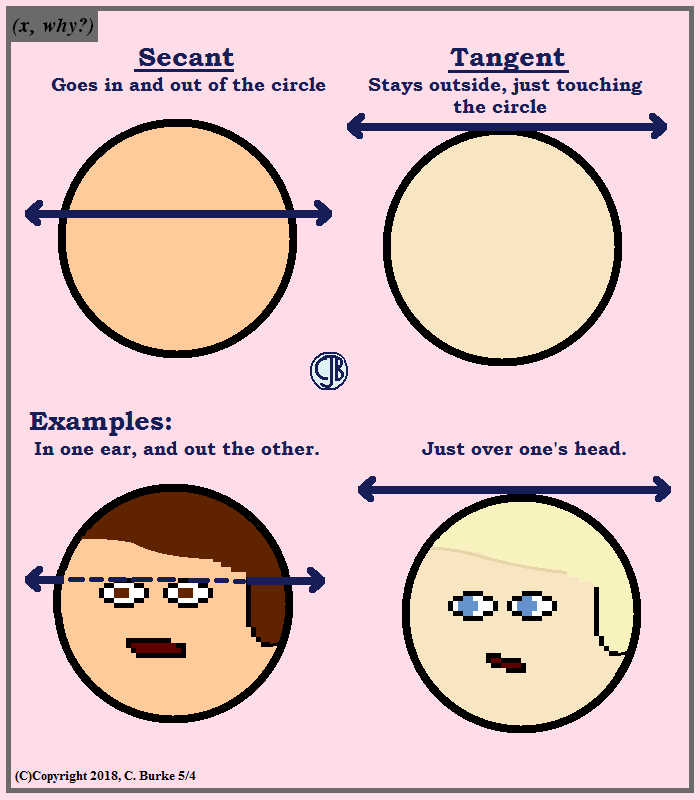The ''in one ear and out the other'' comes up fairly often.

Come back often for more funny math and geeky comics.Thursday, May 03, 2018

Algebra 2 Problems of the Day

Daily Algebra 2 questions and answers.

More Algebra 2 problems.

August 2017, Part I

All Questions in Part I are worth 2 credits. No work need be shown. No partial credit.

10. lridium-192 is an isotope of iridium and has a half-life of 73.83 days. If a laboratory experiment begins with 100 grams of Iridium-192, the number of grams, A, of Iridium-192 present after t days would be A = 100(1/2)(t/73.83).
Which equation approximates the amount of Iridium-192 present after t days?

1) A = 100(73.83/2)t
2) A = 100(1/147.66)t
3) A = 100(0.990656)t
4) A = 100(0.116381)t

100(1/2)(t/73.83) = 100((1/2)(1/73.83)) (t)
(1/2)(1/73.83) = 0.99065551184
So 100(1/2)(t/73.83) = 100(0.990656) (t)

11. The distribution of the diameters of ball bearings made under a given manufacturing process is normally distributed with a mean of 4 cm and a standard deviation of 0.2 cm. What proportion of the ball bearings will have a diameter less than 3.7 cm?

1) 0.0668
2) 0.4332
3) 0.8664
4) 0.9500

You'll find "normCdf" using 2nd VARS, which gives you the DISTRIB menu, where it is option 2.
The 0 is your lower boundary, and 3.7 is your upper boundary. You want everything less than 3.7.
The 4 is your mean, and the 0.2 is your standard deviation.
You will get an answer of 0.066807.

12. A polynomial equation of degree three, p(x), is used to model the volume of a rectangular box. The graph of p(x) has x intercepts at −2, 10, and 14. Which statements regarding p(x) could be true? A. The equation of p(x) = (x − 2)(x + 10)(x + 14). B. The equation of p(x) = −(x + 2)(x − 10)(x − 14). C. The maximum volume occurs when x = 10. D. The maximum volume of the box is approximately 56

1) A and C
2) A and D
3) B and C
4) B and D

The zeroes are -2, 10 and 14, so statement A is incorrect but B could be true.
Since 10 is a zero, it does not make sense that it would also be a maximum value. The line will be either increasing or decreasing as it passes through x = 10. In either case, x = 10 is not a maximum point. This eliminates statement C, so only Choice 4 is left.
Putting p(x) = −(x + 2)(x − 10)(x − 14) into the calculator, you will see that the maximum is 56, so statement 4 could be true if statement 2 is true.

More Algebra 2 problems.

Wednesday, May 02, 2018

Algebra 2 Problems of the Day

Daily Algebra 2 questions and answers.

More Algebra 2 problems.

August 2017, Part I

All Questions in Part I are worth 2 credits. No work need be shown. No partial credit.

7. Which diagram represents an angle, α, measuring 13π / 20 radians drawn in standard position, and its reference angle, θ?

The reference angle is always measured from the x-axis and will not be greater than 90 degrees. In choice 1, the supplement of the reference angle is shown. In choice 3, the measure from the y-axis is shown.

Choices 1, 3, and 4 shown a correct angle measuring 13π / 20 radians. Choice 2 shows a measured in a clockwise, instead of counterclockwise, direction.

8. What are the zeros of P(m) = (m2 - 4)(m2 + 1)?
1) 2 and − 2, only
2) 2, − 2, and − 4
3) −4, i, and − i
4) 2, − 2, i, and − i

Answer: 4) 2, − 2, i, and − i
(m2 - 4) is the difference of two perfect squares and can be factored into two conjugates:
(m + 2)(m - 2), which means that P(m) has zeroes at -2 and 2.
Likewise, if (m2 - 4) = 0
Then m2 = 4
and m = +2

(m2 + 1) has no real roots, but does have imaginary roots.
m2 = -1
m = +(-1)^.5 = +i

9. The value of a new car depreciates over time. Greg purchased a new car in June 2011. The value, V, of his car after t years can be modeled by the equation log0.8( V/17000) = t.
What is the average decreasing rate of change per year of the value of the car from June 2012 to June 2014, to the nearest ten dollars per year??
1) 1960
2) 2180
3) 2450
4) 2770

In 2014, t = 3 years, and in 2012, t = 1 year.
Find the value of the function when t = 3 and t = 1, and then divide by the change in t, which is 3 - 1 = 2.

(17000(0.8)3 - 17000(0.8)1)/(3-1) = -2448 = -2450, to the nearest ten.

More Algebra 2 problems.

Tuesday, May 01, 2018

Algebra 2 Problems of the Day

Daily Algebra 2 questions and answers.

More Algebra 2 problems.

August 2017, Part I

All Questions in Part I are worth 2 credits. No work need be shown. No partial credit.

4. The solution set for the equation sqrt(x + 14) − sqrt(2x + 5) = 1 is
1) {-6}
2) {2}
3) {18}
4) {2,22}

First of all, since this is multiple choice, you can put the following into your calculator:

y = (x + 14)^(1/2) − (2x + 5)^(1/2),
and see which value(s) of x have a y value of 1. This is an easy alternative because all the choices are integers.

To solve algebraically, move one of the radicals to the right side of the equation.
Square both sides to remove the radical from the left side of the equation. The right side will still have a radical.
Move all the rational terms from the right side to the left side.
Square both sides of the equation a second time. Two squared is 4, and the square root of 2x + 5, sqaured, is 2x + 5.
Distribute the 4.
Move all the terms to the left side, leaving a quadratic equation in standard form, equal to zero.
Factor the quadratic, and find both zeroes.

If you were checking the answers, you'll notice that three of the answers only had one value, meaning that your final quadratic equation would have had to have been a perfect square, which this is not. Half of -24 is -12, and (-12) squared is 144, not 44. Just based on this you could have eliminated choices 1, 2 and 3.

5. As x increases from 0 to π/2, the graph of the equation y = 2tanx will
1) increase from 0 to 2
2) decrease from 0 to −2
3) increase without limit
4) decrease without limit

Between 0 and π/2, tangent increases toward infinity. (y = 2tanx is undefined for π/2.)

6. Which equation represents a parabola with the focus at (0,−1) and the directrix of y = 1?
1) x2 = -8y
2) x2 = -4y
3) x2 = 8y
4) x2 = 4y

The distance from the focus to the directrix is 2. The vertex is half-way between the two, at a distance of p = 1. This means that the vertex of the parabola is the origin, (0, 0). (This should be obvious from the choices.)

Using the equation: y = -1/(4p)(x - h)2 + k, substitute what we know:
y = -1/(4(1))(x - 0)2 + 0
y = -1/4 x2,
which is the same as -4y = x2.